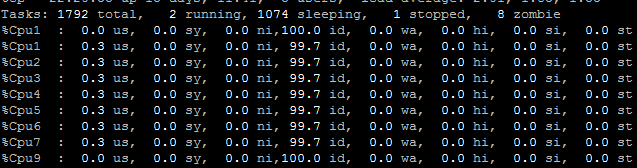# The difference between OpenBLAS and MKL

I want to compile the source code in ARMv8 machine, and MKL does not support ARM.Then, I choose OpenBLAS as the BLAS.However, when I compile it successfully, I found the CPU utilization is low.My machine has 128 cores but when I run the following benchmark:

``````import timeit
runtimes = []
threads =  + [t for t in range(2, 49, 2)]
r = timeit.timeit(setup = "import torch; x = torch.randn(1024, 1024); y = torch.randn(1024, 1024)", stmt="torch.mm(x, y)", number=100)
runtimes.append(r)
# ... plotting (threads, runtimes) ...
``````

The CPU untilzation just like this and time has hardly changed.The compile step just like this:
export USE_OPENMP=1
export BLAS=OpenBLAS
export USE_CUDA=0
python setup.py install

machine:aarch64

Can OpenBLAS do parallel computing on Pytorch?If not, how can I do to improve the performance?